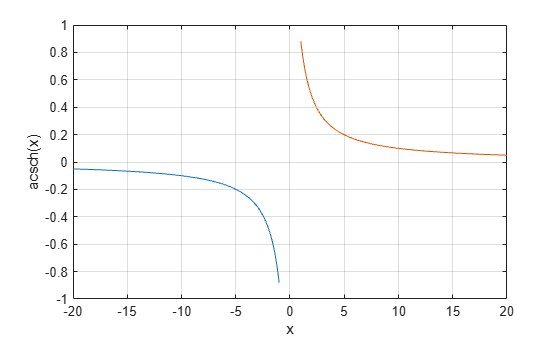# acsch

Inverse hyperbolic cosecant

## Syntax

``Y = acsch(X)``

## Description

example

````Y = acsch(X)` returns the inverse hyperbolic cosecant of the elements of `X`. The function accepts both real and complex inputs. All angles are in radians.```

## Examples

collapse all

Find the inverse hyperbolic cosecant of the elements of vector `X`. The `acsch` function acts on `X` element-wise.

```X = [2 -3 1+2i]; Y = acsch(X)```
```Y = 1×3 complex 0.4812 + 0.0000i -0.3275 + 0.0000i 0.2156 - 0.4016i ```

Plot the inverse hyperbolic cosecant function over the intervals $-20\le x\le -1$ and $1\le x\le 20$.

```x1 = -20:0.01:-1; x2 = 1:0.01:20; plot(x1,acsch(x1),x2,acsch(x2)) grid on xlabel('x') ylabel('acsch(x)')```## Input Arguments

collapse all

Hyperbolic cosecant of angle, specified as a scalar, vector, matrix, or multidimensional array. The `acsch` operation is element-wise when `X` is nonscalar.

Data Types: `single` | `double`
Complex Number Support: Yes

collapse all

### Inverse Hyperbolic Cosecant

For real values $x$ in the domain $x<0$ and $x>0$, the inverse hyperbolic cosecant satisfies

`${\text{csch}}^{-1}\left(z\right)={\mathrm{sinh}}^{-1}\left(\frac{1}{z}\right)=\mathrm{log}\left(\frac{1}{x}+\sqrt{\frac{1}{{x}^{2}}+1}\right).$`

For complex numbers $z=x+iy$, the call `acsch(z)` returns complex results.